Courses

# Fields - Field Theory Mathematics Notes | EduRev

## Algebra for IIT JAM Mathematics

Created by: Veda Institute

## Mathematics : Fields - Field Theory Mathematics Notes | EduRev

The document Fields - Field Theory Mathematics Notes | EduRev is a part of the Mathematics Course Algebra for IIT JAM Mathematics.
All you need of Mathematics at this link: Mathematics

In abstract algebra, a field is an algebraic structure with notions of addition, subtraction, multiplication, and division, satisfying certain axioms. The most commonly used fields are the field of real numbers, the field of complex numbers, and the field of rational numbers, but there are also finite fields, fields of functions, various algebraic number fields, p-adic fields, and so forth.

Any field may be used as the scalars for a vector space, which is the standard general context for linear algebra. The theory of field extensions (including Galois theory) involves the roots of polynomials with coefficients in a field; among other results, this theory leads to impossibility proofs for the classical problems of angle trisection and squaring the circle with a compass and straightedge, as well as a proof of the Abel–Ruffini theorem on the algebraic insolubility of quintic equations. In modern mathematics, the theory of fields (or field theory) plays an essential role in number theory and algebraic geometry.

As an algebraic structure, every field is a ring, but not every ring is a field. The most important difference is that fields allow for division (though not division by zero), while a ring need not possess multiplicative inverses. Also, the multiplication operation in a field is required to be commutative. A ring in which division is possible but commutativity is not assumed (such as the quaternions) is called a division ring or skew field. (Historically, division rings were sometimes referred to as fields, while fields were called “commutative fields”.)

As a ring, a field may be classified as a specific type of integral domain, and can be characterized by the following (not exhaustive) chain of class inclusions:

Commutative rings ⊃ integral domains ⊃ integrally closed domains ⊃ unique factorization domains ⊃ principal ideal domains ⊃ Euclidean domains ⊃ fields ⊃ finite fields.

Definition and illustration

Intuitively, a field is a set F that is a commutative group with respect to two compatible operations, addition and multiplication, with "compatible" being formalized by distributivity, and the caveat that the additive identity (0) has no multiplicative inverse (one cannot divide by 0).

The most common way to formalize this is by defining a field as a set together with two operations, usually called addition and multiplication, and denoted by + and ·, respectively, such that the following axioms hold; subtraction and division are defined implicitly in terms of the inverse operations of addition and multiplication:

Closure of F under addition and multiplication

For all a, b in F, both a + b and a · b are in F (or more formally, + and · are binary operations on F).

For all a and b in F, the following equalities hold: a + b = b + a and a · b = b · a.

There exists an element of F, called the additive identity element and denoted by 0, such that for all a in F, a + 0 = a. Likewise, there is an element, called the multiplicative identity element and denoted by 1, such that for all a in F, a · 1 = a. For technical reasons, the additive identity and the multiplicative identity are required to be distinct.

For every a in F, there exists an element −a in F, such that a + (−a) = 0. Similarly, for any a in F other than 0, there exists an element a−1 in F, such that a · a−1 = 1. (The elements a + (−b) and a · b−1 are also denoted a − b and a/b, respectively.) In other words, subtraction and division operations exist.

For all a, b and c in F, the following equality holds: a · (b + c) = (a · b) + (a · c).

Note that all but the last axiom are exactly the axioms for a commutative group, while the last axiom is a compatibility condition between the two operations.

First example: rational numbers

A simple example of a field is the field of rational numbers, consisting of the fractions a/b, where a and b are integers, and b ≠ 0. The additive inverse of such a fraction is simply −a/b, and the multiplicative inverse—provided that a ≠ 0, as well—is b/a. To see the latter, note that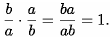The abstractly required field axioms reduce to standard properties of rational numbers, such as the law of distributivity or the law of commutativity and law of associativity.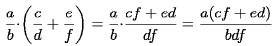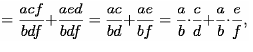Second example: a field with four elements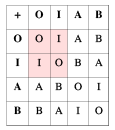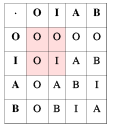In addition to familiar number systems such as the rationals, there are other, less immediate examples of fields. The following example is a field consisting of four elements called O, I, A and B. The notation is chosen such that O plays the role of the additive identity element (denoted 0 in the axioms), and I is the multiplicative identity (denoted 1 above). One can check that all field axioms are satisfied. For example:

A · (B + A) = A · I = A, which equals A · B + A · A = I + B = A, as required by the distributivity.

The above field is called a finite field with four elements, and can be denoted F4. Field theory is concerned with understanding the reasons for the existence of this field, defined in a fairly ad-hoc manner, and describing its inner structure. For example, from a glance at the multiplication table, it can be seen that any non-zero element, i.e., I, A, and B, is a power of A: A = A1, B = A2 = A · A, and finally I = A3 = A · A · A. This is not a coincidence, but rather one of the starting points of a deeper understanding of (finite) fields.

Alternative axiomatizations

As with other algebraic structures, there exist alternative axiomatizations. Because of the relations between the operations, one can alternatively axiomatize a field by explicitly assuming that are four binary operations (add, subtract, multiply, divide) with axioms relating these, or in terms of two binary operations (add, multiply) and two unary operations (additive inverse, multiplicative inverse), or other variants.

The usual axiomatization in terms of the two operations of addition and multiplication is brief and allows the other operations to be defined in terms of these basic ones, but in other contexts, such as topology and category theory, it is important to include all operations as explicitly given, rather than implicitly defined (compare topological group).
This is because without further assumptions, the implicitly defined inverses may not be continuous (in topology), or may not be able to be defined (in category theory): defining an inverse requires that one be working with a set, not a more general object.

Related algebraic structures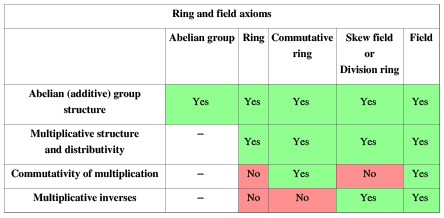The axioms imposed above resemble the ones familiar from other algebraic structures. For example, the existence of the binary operation "·", together with its commutativity, associativity, (multiplicative) identity element and inverses are precisely the axioms for an abelian group. In other words, for any field, the subset of nonzero elements F \ {0}, also often denoted F×, is an abelian group (F×,·) usually called multiplicative group of the field. Likewise (F, +) is an abelian group. The structure of a field is hence the same as specifying such two group structures (on the same set), obeying the distributivity.

Important other algebraic structures such as rings arise when requiring only part of the above axioms. For example, if the requirement of commutativity of the multiplication operation · is dropped, one gets structures usually called division rings or skew fields.

Remarks

By elementary group theory, applied to the abelian groups (F×, ·), and (F, +), the additive inverse −a and the multiplicative inverse a−1 are uniquely determined by a.

Similar direct consequences from the field axioms include

−(a · b) = (−a) · b = a · (−b), in particular −a = (−1) · a as well

as

a · 0 = 0.

Both can be shown by replacing b or c with 0 in the distributive property

History

The concept of field was used implicitly by Niels Henrik Abel and Évariste Galois in their work on the solvability of polynomial equations with rational coefficients of degree 5 or higher.

In 1857 Karl von Staudt published his Algebra of Throws which provided a geometric model satisfying the axioms of a field. This construction has been frequently recalled as a contribution to the foundations of mathematics.

In 1871, Richard Dedekind introduced, for a set of real or complex numbers which is closed under the four arithmetic operations, the German word Körper, which means "body" or "corpus" (to suggest an organically closed entity), hence the common use of the letter K to denote a field. He also defined rings (then called order or order-modul), but the term "a ring" (Zahlring) was invented by Hilbert. In 1893, Eliakim Hastings Moore called the concept "field" in English.

In 1881, Leopold Kronecker defined what he called a "domain of rationality", which is indeed a field of polynomials in modern terms. In 1893, Heinrich M. Weber gave the first clear definition of an abstract field. In 1910 Ernst Steinitz published the very influential paper Algebraische Theorie der Körper (English: Algebraic Theory of Fields). In this paper he axiomatically studies the properties of fields and defines many important field theoretic concepts like prime field, perfect field and the transcendence degree of a field extension.

Emil Artin developed the relationship between groups and fields in great detail during 1928-1942.

Examples

Rationals and algebraic numbers

The field of rational numbers Q has been introduced above. A related class of fields very important in number theory are algebraic number fields. We will first give an example, namely the field Q(ζ) consisting of numbers of the form

a + bζ

with a, b ∈ Q, where ζ is a primitive third root of unity, i.e., a complex number satisfying ζ3 = 1, ζ ≠ 1. This field extension can be used to prove a special case of Fermat's last theorem, which asserts the non-existence of rational nonzero solutions to the equation

x3 + y3 = z3.

In the language of field extensions detailed below, Q(ζ) is a field extension of degree 2. Algebraic number fields are by definition finite field extensions of Q, that is, fields containing Q having finite dimension as a Q-vector space.

Reals, complex numbers, and p-adic numbers

Take the real numbers R, under the usual operations of addition and multiplication. When the real numbers are given the usual ordering, they form a complete ordered field; it is this structure which provides the foundation for most formal treatments of calculus.

The complex numbers C consist of expressions

a + bi

where i is the imaginary unit, i.e., a (non-real) number satisfying i2 = −1. Addition and multiplication of real numbers are defined in such a way that all field axioms hold for C. For example, the distributive law enforces

(a + bi)·(c + di) = ac + bci + adi + bdi2, which equals ac−bd + (bc + ad)i.

The real numbers can be constructed by completing the rational numbers, i.e., filling the "gaps": for example √2 is such a gap. By a formally very similar procedure, another important class of fields, the field of p-adic numbers Qp is built. It is used in number theory and p-adic analysis.

Hyperreal numbers and superreal numbers extend the real numbers with the addition of infinitesimal and infinite numbers.

Constructible numbers

In antiquity, several geometric problems concerned the (in)feasibility of constructing certain numbers with compass and straightedge. For example it was unknown to the Greeks that it is in general impossible to trisect a given angle. Using the field notion and field theory allows these problems to be settled. To do so, the field of constructible numbers is considered. It contains, on the plane, the points 0 and 1, and all complex numbers that can be constructed from these two by a finite number of construction steps using only compass and straightedge. This set, endowed with the usual addition and multiplication of complex numbers does form a field. For example, multiplying two (real) numbers r1 and r2 that have already been constructed can be done using construction at the right, based on the intercept theorem. This way, the obtained field F contains all rational numbers, but is bigger than Q, because for any f ∈ F, the square root of f is also a constructible number.
built. It is used in number theory and p-adic analysis.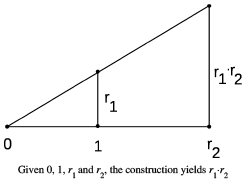Offer running on EduRev: Apply code STAYHOME200 to get INR 200 off on our premium plan EduRev Infinity!

58 docs

,

,

,

,

,

,

,

,

,

,

,

,

,

,

,

,

,

,

,

,

,

;